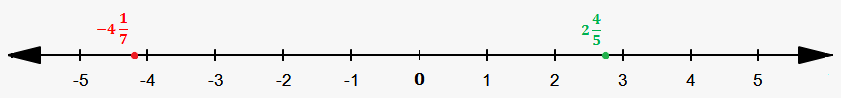# Mixed Numbers on a Number Line

So, where are mixed numbers located on a number line?

For example, where is ${2}\frac{{4}}{{5}}$ located? We see that this number consists of integer 2 and proper fraction $\frac{{4}}{{5}}$.

This means that it is greater than 2 (because there is 2 and proper fraction), but it is less than 2+1=3 because proper fraction is less than 1.

This means that ${2}\frac{{4}}{{5}}$ is between 2 and 3.

Let's see how this works with negative numbers.

For example, consider $-{4}\frac{{1}}{{7}}$.

It is less than -4 because there is -4 and proper fraction (which is also negative because minus is in front of mixed number), but it is greater than -4-1=-5, because, since positive proper fraction is less than 1 then negative proper fraction is greater than -1.